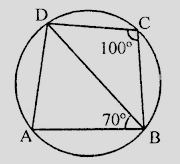"
">

# In the figure, $ABCD$ is a cyclic quadrilateral. If $\angle BCD = 100^o$ and $\angle ABD = 70^o$, find $\angle ADB$."

Given:

In the figure, $ABCD$ is a cyclic quadrilateral.

$\angle BCD = 100^o$ and $\angle ABD = 70^o$.

To do:

We have to find $\angle ADB$.

Solution:

$ABCD$ is a cyclic quadrilateral.

This implies,

$\angle A + \angle C = 180^o$                 (Sum of opposite angles)

$\angle A + 100^o = 180^o$

$\angle A = 180^o- 100^o = 80^o$

In $\triangle ABD$,

$\angle A + \angle ABD + \angle ADB = 180^o$

$80^o + 70^o + \angle ADB = 180^o$

$150^o +\angle ADB = 180^o$

$\angle ADB = 180^o- 150^o = 30^o$

Hence $\angle ADB = 30^o$.

Updated on: 10-Oct-2022

26 Views